# An Intuitive Understanding of Word Embeddings: From Count Vectors to Word2Vec

## Introduction

Before we start, have a look at the below examples.

1. You open Google and search for a news article on the ongoing Champions trophy and get hundreds of search results in return about it.
2. Nate silver analysed millions of tweets and correctly predicted the results of 49 out of 50 states in 2008 U.S Presidential Elections.
3. You type a sentence in google translate in English and get an Equivalent Chinese conversion.So what do the above examples have in common?

You possible guessed it right – TEXT processing. All the above three scenarios deal with humongous amount of text to perform different range of tasks like clustering in the google search example, classification in the second and Machine Translation in the third.

Humans can deal with text format quite intuitively but provided we have millions of documents being generated in a single day, we cannot have humans performing the above the three tasks. It is neither scalable nor effective.So, how do we make computers of today perform clustering, classification etc on a text data since we know that they are generally inefficient at handling and processing strings or texts for any fruitful outputs?

Sure, a computer can match two strings and tell you whether they are same or not. But how do we make computers tell you about football or Ronaldo when you search for Messi? How do you make a computer understand that “Apple” in “Apple is a tasty fruit” is a fruit that can be eaten and not a company?

The answer to the above questions lie in creating a representation for words that capture their meanings, semantic relationships and the different types of contexts they are used in.

And all of these are implemented by using Word Embeddings or numerical representations of texts so that computers may handle them.

Below, we will see formally what are Word Embeddings and their different types and how we can actually implement them to perform the tasks like returning efficient Google search results.

## Problem Statement

The objective of this task is to detect hate speech in tweets. For the sake of simplicity, we say a tweet contains hate speech if it has a racist or sexist sentiment associated with it. So, the task is to classify racist or sexist tweets from other tweets.

Formally, given a training sample of tweets and labels, where label ‘1’ denotes the tweet is racist/sexist and label ‘0’ denotes the tweet is not racist/sexist, your objective is to predict the labels on the test dataset.

Practice Now

1. What are Word Embeddings?
2. Different types of Word Embeddings
2.1  Frequency based Embedding
2.1.1 Count Vectors
2.1.2 TF-IDF
2.1.3 Co-Occurrence Matrix
2.2  Prediction based Embedding
2.2.1 CBOW
2.2.2 Skip-Gram
3. Word Embeddings use case scenarios(what all can be done using word embeddings? eg: similarity, odd one out etc.)
4. Using pre-trained Word Vectors
5. Training your own Word Vectors
6. End Notes

## 1. What are Word Embeddings?

In very simplistic terms, Word Embeddings are the texts converted into numbers and there may be different numerical representations of the same text. But before we dive into the details of Word Embeddings, the following question should be asked – Why do we need Word Embeddings?

As it turns out, many Machine Learning algorithms and almost all Deep Learning Architectures are incapable of processing strings or plain text in their raw form. They require numbers as inputs to perform any sort of job, be it classification, regression etc. in broad terms. And with the huge amount of data that is present in the text format, it is imperative to extract knowledge out of it and build applications. Some real world applications of text applications are – sentiment analysis of reviews by Amazon etc., document or news classification or clustering by Google etc.

Let us now define Word Embeddings formally. A Word Embedding format generally tries to map a word using a dictionary to a vector. Let us break this sentence down into finer details to have a clear view.

Take a look at this example – sentence=” Word Embeddings are Word converted into numbers ”

A word in this sentence may be “Embeddings” or “numbers ” etc.

A dictionary may be the list of all unique words in the sentence. So, a dictionary may look like – [‘Word’,’Embeddings’,’are’,’Converted’,’into’,’numbers’]

A vector representation of a word may be a one-hot encoded vector where 1 stands for the position where the word exists and 0 everywhere else. The vector representation of “numbers” in this format according to the above dictionary is [0,0,0,0,0,1] and of converted is[0,0,0,1,0,0].

This is just a very simple method to represent a word in the vector form. Let us look at different types of Word Embeddings or Word Vectors and their advantages and disadvantages over the rest.

## 2. Different types of Word Embeddings

The different types of word embeddings can be broadly classified into two categories-

1. Frequency based Embedding
2. Prediction based Embedding

Let us try to understand each of these methods in detail.

### 2.1 Frequency based Embedding

There are generally three types of vectors that we encounter under this category.

1. Count Vector
2. TF-IDF Vector
3. Co-Occurrence Vector

Let us look into each of these vectorization methods in detail.

#### 2.1.1 Count Vector

Consider a Corpus C of D documents {d1,d2…..dD} and N unique tokens extracted out of the corpus C. The N tokens will form our dictionary and the size of the Count Vector matrix M will be given by D X N. Each row in the matrix M contains the frequency of tokens in document D(i).

Let us understand this using a simple example.

D1: He is a lazy boy. She is also lazy.

D2: Neeraj is a lazy person.

The dictionary created may be a list of unique tokens(words) in the corpus =[‘He’,’She’,’lazy’,’boy’,’Neeraj’,’person’]

Here, D=2, N=6

The count matrix M of size 2 X 6 will be represented as –

 He She lazy boy Neeraj person D1 1 1 2 1 0 0 D2 0 0 1 0 1 1

Now, a column can also be understood as word vector for the corresponding word in the matrix M. For example, the word vector for ‘lazy’ in the above matrix is [2,1] and so on.Here, the rows correspond to the documents in the corpus and the columns correspond to the tokens in the dictionary. The second row in the above matrix may be read as – D2 contains ‘lazy’: once, ‘Neeraj’: once and ‘person’ once.

Now there may be quite a few variations while preparing the above matrix M. The variations will be generally in-

1. The way dictionary is prepared.
Why? Because in real world applications we might have a corpus which contains millions of documents. And with millions of document, we can extract hundreds of millions of unique words. So basically, the matrix that will be prepared like above will be a very sparse one and inefficient for any computation. So an alternative to using every unique word as a dictionary element would be to pick say top 10,000 words based on frequency and then prepare a dictionary.
2. The way count is taken for each word.
We may either take the frequency (number of times a word has appeared in the document) or the presence(has the word appeared in the document?) to be the entry in the count matrix M. But generally, frequency method is preferred over the latter.

Below is a representational image of the matrix M for easy understanding.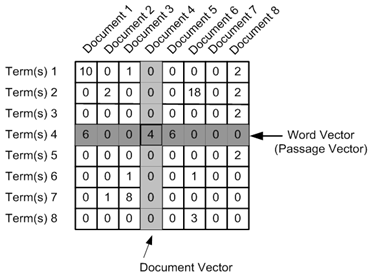#### 2.1.2 TF-IDF vectorization

This is another method which is based on the frequency method but it is different to the count vectorization in the sense that it takes into account not just the occurrence of a word in a single document but in the entire corpus. So, what is the rationale behind this? Let us try to understand.

Common words like ‘is’, ‘the’, ‘a’ etc. tend to appear quite frequently in comparison to the words which are important to a document. For example, a document A on Lionel Messi is going to contain more occurences of the word “Messi” in comparison to other documents. But common words like “the” etc. are also going to be present in higher frequency in almost every document.

Ideally, what we would want is to down weight the common words occurring in almost all documents and give more importance to words that appear in a subset of documents.

TF-IDF works by penalising these common words by assigning them lower weights while giving importance to words like Messi in a particular document.

So, how exactly does TF-IDF work?

Consider the below sample table which gives the count of terms(tokens/words) in two documents.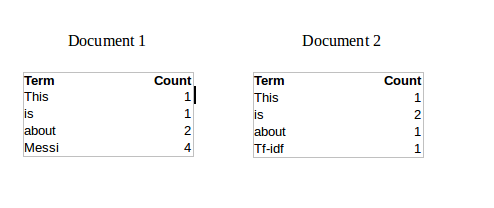Now, let us define a few terms related to TF-IDF.

TF = (Number of times term t appears in a document)/(Number of terms in the document)

So, TF(This,Document1) = 1/8

TF(This, Document2)=1/5

It denotes the contribution of the word to the document i.e words relevant to the document should be frequent. eg: A document about Messi should contain the word ‘Messi’ in large number.

IDF = log(N/n), where, N is the number of documents and n is the number of documents a term t has appeared in.

where N is the number of documents and n is the number of documents a term t has appeared in.

So, IDF(This) = log(2/2) = 0.

So, how do we explain the reasoning behind IDF? Ideally, if a word has appeared in all the document, then probably that word is not relevant to a particular document. But if it has appeared in a subset of documents then probably the word is of some relevance to the documents it is present in.

Let us compute IDF for the word ‘Messi’.

IDF(Messi) = log(2/1) = 0.301.

Now, let us compare the TF-IDF for a common word ‘This’ and a word ‘Messi’ which seems to be of relevance to Document 1.

TF-IDF(This,Document1) = (1/8) * (0) = 0

TF-IDF(This, Document2) = (1/5) * (0) = 0

TF-IDF(Messi, Document1) = (4/8)*0.301 = 0.15

As, you can see for Document1 , TF-IDF method heavily penalises the word ‘This’ but assigns greater weight to ‘Messi’. So, this may be understood as ‘Messi’ is an important word for Document1 from the context of the entire corpus.

#### 2.1.3 Co-Occurrence Matrix with a fixed context window

The big idea – Similar words tend to occur together and will have similar context for example – Apple is a fruit. Mango is a fruit.
Apple and mango tend to have a similar context i.e fruit.

Before I dive into the details of how a co-occurrence matrix is constructed, there are two concepts that need to be clarified – Co-Occurrence and Context Window.

Co-occurrence – For a given corpus, the co-occurrence of a pair of words say w1 and w2 is the number of times they have appeared together in a Context Window.

Context Window – Context window is specified by a number and the direction. So what does a context window of 2 (around) means? Let us see an example below,

 Quick Brown Fox Jump Over The Lazy Dog

The green words are a 2 (around) context window for the word ‘Fox’ and for calculating the co-occurrence only these words will be counted. Let us see context window for the word ‘Over’.

 Quick Brown Fox Jump Over The Lazy Dog

Now, let us take an example corpus to calculate a co-occurrence matrix.

Corpus = He is not lazy. He is intelligent. He is smart.

 He is not lazy intelligent smart He 0 4 2 1 2 1 is 4 0 1 2 2 1 not 2 1 0 1 0 0 lazy 1 2 1 0 0 0 intelligent 2 2 0 0 0 0 smart 1 1 0 0 0 0

Let us understand this co-occurrence matrix by seeing two examples in the table above. Red and the blue box.

Red box- It is the number of times ‘He’ and ‘is’ have appeared in the context window 2 and it can be seen that the count turns out to be 4. The below table will help you visualise the count.

 He is not lazy He is intelligent He is smart He is not lazy He is intelligent He is smart He is not lazy He is intelligent He is smart He is not lazy He is intelligent He is smart

while the word ‘lazy’ has never appeared with ‘intelligent’ in the context window and therefore has been assigned 0 in the blue box.

Variations of Co-occurrence Matrix

Let’s say there are V unique words in the corpus. So Vocabulary size = V. The columns of the Co-occurrence matrix form the context words. The different variations of Co-Occurrence Matrix are-

1. A co-occurrence matrix of size V X V. Now, for even a decent corpus V gets very large and difficult to handle. So generally, this architecture is never preferred in practice.
2. A co-occurrence matrix of size V X N where N is a subset of V and can be obtained by removing irrelevant words like stopwords etc. for example. This is still very large and presents computational difficulties.

But, remember this co-occurrence matrix is not the word vector representation that is generally used. Instead, this Co-occurrence matrix is decomposed using techniques like PCA, SVD etc. into factors and combination of these factors forms the word vector representation.

Let me illustrate this more clearly. For example, you perform PCA on the above matrix of size VXV. You will obtain V principal components. You can choose k components out of these V components. So, the new matrix will be of the form V X k.

And, a single word, instead of being represented in V dimensions will be represented in k dimensions while still capturing almost the same semantic meaning. k is generally of the order of hundreds.

So, what PCA does at the back is decompose Co-Occurrence matrix into three matrices, U,S and V where U and V are both orthogonal matrices. What is of importance is that dot product of U and S gives the word vector representation and V gives the word context representation.1. It preserves the semantic relationship between words. i.e man and woman tend to be closer than man and apple.
2. It uses SVD at its core, which produces more accurate word vector representations than existing methods.
3. It uses factorization which is a well-defined problem and can be efficiently solved.
4. It has to be computed once and can be used anytime once computed. In this sense, it is faster in comparison to others.

1. It requires huge memory to store the co-occurrence matrix.
But, this problem can be circumvented by factorizing the matrix out of the system for example in Hadoop clusters etc. and can be saved.

## 2.2 Prediction based Vector

Pre-requisite: This section assumes that you have a working knowledge of how a neural network works and the mechanisms by which weights in an NN are updated. If you are new to Neural Network, I would suggest you go through this awesome article by Sunil to gain a very good understanding of how NN works.

So far, we have seen deterministic methods to determine word vectors. But these methods proved to be limited in their word representations until Mitolov etc. el introduced word2vec to the NLP community. These methods were prediction based in the sense that they provided probabilities to the words and proved to be state of the art for tasks like word analogies and word similarities. They were also able to achieve tasks like King -man +woman = Queen, which was considered a result almost magical. So let us look at the word2vec model used as of today to generate word vectors.

Word2vec is not a single algorithm but a combination of two techniques – CBOW(Continuous bag of words) and Skip-gram model. Both of these are shallow neural networks which map word(s) to the target variable which is also a word(s). Both of these techniques learn weights which act as word vector representations. Let us discuss both these methods separately and gain intuition into their working.

### 2.2.1 CBOW (Continuous Bag of words)

The way CBOW work is that it tends to predict the probability of a word given a context. A context may be a single word or a group of words. But for simplicity, I will take a single context word and try to predict a single target word.

Suppose, we have a corpus C = “Hey, this is sample corpus using only one context word.” and we have defined a context window of 1. This corpus may be converted into a training set for a CBOW model as follow. The input is shown below. The matrix on the right in the below image contains the one-hot encoded from of the input on the left.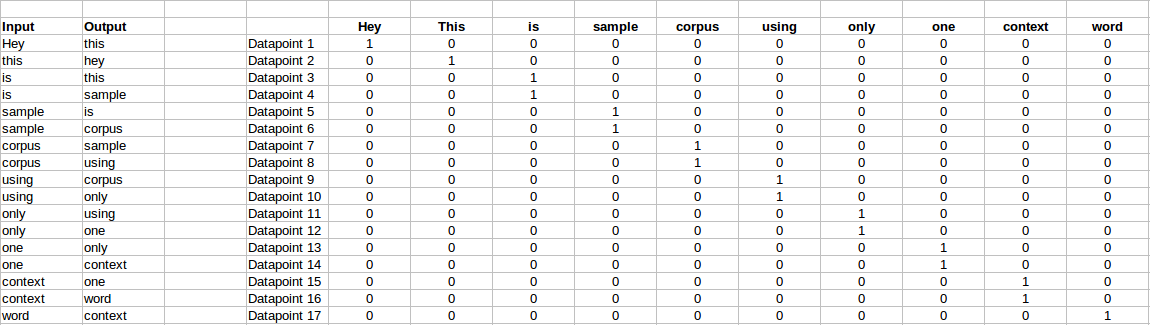The target for a single datapoint say Datapoint 4 is shown as below

 Hey this is sample corpus using only one context word 0 0 0 1 0 0 0 0 0 0

This matrix shown in the above image is sent into a shallow neural network with three layers: an input layer, a hidden layer and an output layer. The output layer is a softmax layer which is used to sum the probabilities obtained in the output layer to 1. Now let us see how the forward propagation will work to calculate the hidden layer activation.

Let us first see a diagrammatic representation of the CBOW model.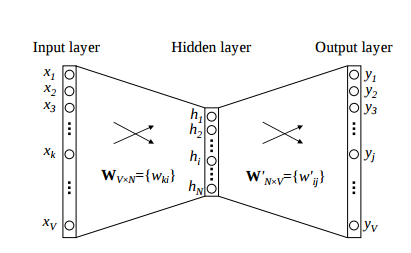The matrix representation of the above image for a single data point is below.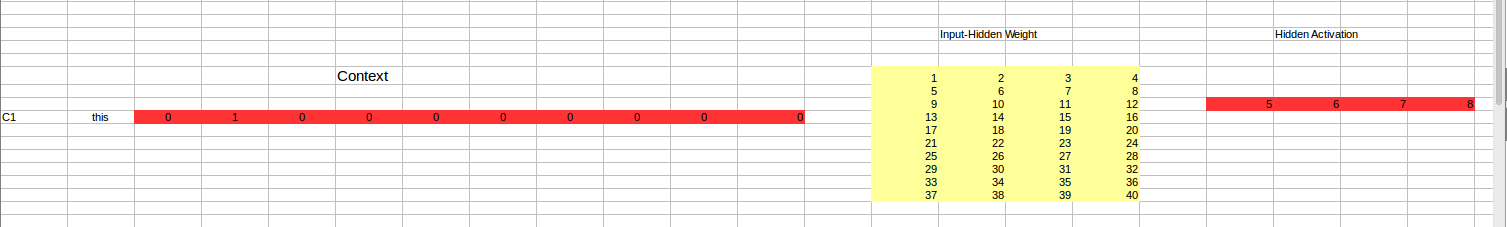The flow is as follows:

1. The input layer and the target, both are one- hot encoded of size [1 X V]. Here V=10 in the above example.
2. There are two sets of weights. one is between the input and the hidden layer and second between hidden and output layer.
Input-Hidden layer matrix size =[V X N] , hidden-Output layer matrix  size =[N X V] : Where N is the number of dimensions we choose to represent our word in. It is arbitary and a hyper-parameter for a Neural Network. Also, N is the number of neurons in the hidden layer. Here, N=4.
3. There is a no activation function between any layers.( More specifically, I am referring to linear activation)
4. The input is multiplied by the input-hidden weights and called hidden activation. It is simply the corresponding row in the input-hidden matrix copied.
5. The hidden input gets multiplied by hidden- output weights and output is calculated.
6. Error between output and target is calculated and propagated back to re-adjust the weights.
7. The weight  between the hidden layer and the output layer is taken as the word vector representation of the word.

We saw the above steps for a single context word. Now, what about if we have multiple context words? The image below describes the architecture for multiple context words.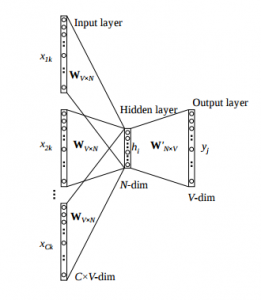Below is a matrix representation of the above architecture for an easy understanding.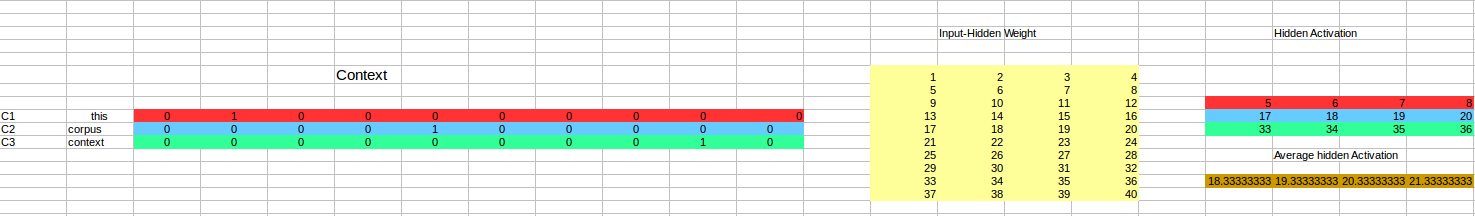The image above takes 3 context words and predicts the probability of a target word. The input can be assumed as taking three one-hot encoded vectors in the input layer as shown above in red, blue and green.

So, the input layer will have 3 [1 X V] Vectors in the input as shown above and 1 [1 X V] in the output layer. Rest of the architecture is same as for a 1-context CBOW.

The steps remain the same, only the calculation of hidden activation changes. Instead of just copying the corresponding rows of the input-hidden weight matrix to the hidden layer, an average is taken over all the corresponding rows of the matrix. We can understand this with the above figure. The average vector calculated becomes the hidden activation. So, if we have three context words for a single target word, we will have three initial hidden activations which are then averaged element-wise to obtain the final activation.

In both a single context word and multiple context word, I have shown the images till the calculation of the hidden activations since this is the part where CBOW differs from a simple MLP network. The steps after the calculation of hidden layer are same as that of the MLP as mentioned in this article – Understanding and Coding Neural Networks from scratch.

The differences between MLP and CBOW are  mentioned below for clarification:

1. The objective function in MLP is a MSE(mean square error) whereas in CBOW it is negative log likelihood of a word given a set of context i.e -log(p(wo/wi)), where p(wo/wi) is given as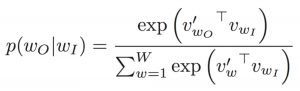wo : output word
wi: context words

2. The gradient of error with respect to hidden-output weights and input-hidden weights are different since MLP has  sigmoid activations(generally) but CBOW has linear activations. The method however to calculate the gradient is same as an MLP.

1. Being probabilistic is nature, it is supposed to perform superior to deterministic methods(generally).
2. It is low on memory. It does not need to have huge RAM requirements like that of co-occurrence matrix where it needs to store three huge matrices.

1. CBOW takes the average of the context of a word (as seen above in calculation of hidden activation). For example, Apple can be both a fruit and a company but CBOW takes an average of both the contexts and places it in between a cluster for fruits and companies.
2. Training a CBOW from scratch can take forever if not properly optimized.

## 2.2.2 Skip – Gram model

Skip – gram follows the same topology as of CBOW. It just flips CBOW’s architecture on its head. The aim of skip-gram is to predict the context given a word. Let us take the same corpus that we built our CBOW model on. C=”Hey, this is sample corpus using only one context word.” Let us construct the training data.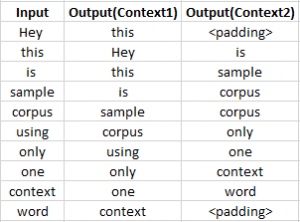The input vector for skip-gram is going to be similar to a 1-context CBOW model. Also, the calculations up to hidden layer activations are going to be the same. The difference will be in the target variable. Since we have defined a context window of 1 on both the sides, there will be “two” one hot encoded target variables and “two” corresponding outputs as can be seen by the blue section in the image.

Two separate errors are calculated with respect to the two target variables and the two error vectors obtained are added element-wise to obtain a final error vector which is propagated back to update the weights.

The weights between the input and the hidden layer are taken as the word vector representation after training. The loss function or the objective is of the same type as of the CBOW model.

The skip-gram architecture is shown below.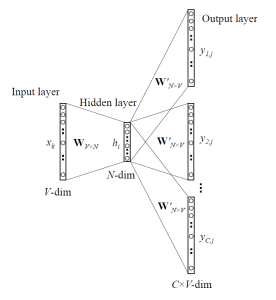For a better understanding, matrix style structure with calculation has been shown below.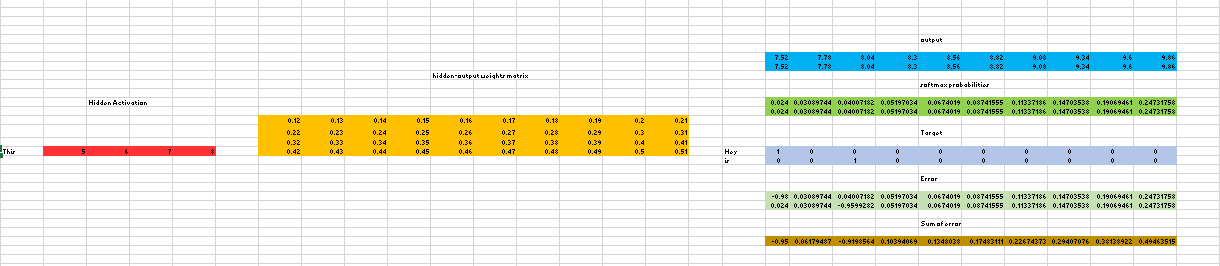Let us break down the above image.

Input layer  size – [1 X V], Input hidden weight matrix size – [V X N], Number of neurons in hidden layer – N, Hidden-Output weight matrix size – [N X V], Output layer size – C [1 X V]

In the above example, C is the number of context words=2, V= 10, N=4

1. The row in red is the hidden activation corresponding to the input one-hot encoded vector. It is basically the corresponding row of input-hidden matrix copied.
2. The yellow matrix is the weight between the hidden layer and the output layer.
3. The blue matrix is obtained by the matrix multiplication of hidden activation and the hidden output weights. There will be two rows calculated for two target(context) words.
4. Each row of the blue matrix is converted into its softmax probabilities individually as shown in the green box.
5. The grey matrix contains the one hot encoded vectors of the two context words(target).
6. Error is calculated by substracting the first row of the grey matrix(target) from the first row of the green matrix(output) element-wise. This is repeated for the next row. Therefore, for target context words, we will have n error vectors.
7. Element-wise sum is taken over all the error vectors to obtain a final error vector.
8. This error vector is propagated back to update the weights.

1. Skip-gram model can capture two semantics for a single word. i.e it will have two vector representations of Apple. One for the company and other for the fruit.
2. Skip-gram with negative sub-sampling outperforms every other method generally.

This is an excellent interactive tool to visualise CBOW and skip gram in action. I would suggest you to really go through this link for a better understanding.

## 3. Word Embeddings use case scenarios

Since word embeddings or word Vectors are numerical representations of contextual similarities between words, they can be manipulated and made to perform amazing tasks like-

1. Finding the degree of similarity between two words.
`model.similarity('woman','man')`
`0.73723527`
2. Finding odd one out.
`model.doesnt_match('breakfast cereal dinner lunch';.split())`
`'cereal'`
3. Amazing things like woman+king-man =queen
`model.most_similar(positive=['woman','king'],negative=['man'],topn=1)`
`queen: 0.508`
4. Probability of a text under the model
`model.score(['The fox jumped over the lazy dog'.split()])`
`0.21`

Below is one interesting visualisation of word2vec.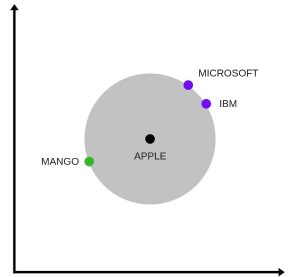The above image is a t-SNE representation of word vectors in 2 dimension and you can see that two contexts of apple have been captured. One is a fruit and the other company.

5.  It can be used to perform Machine Translation.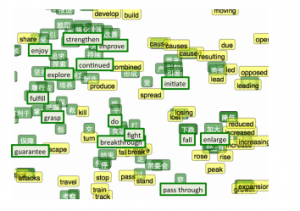The above graph is a bilingual embedding with chinese in green and english in yellow. If we know the words having similar meanings in chinese and english, the above bilingual embedding can be used to translate one language into the other.

## 4. Using pre-trained word vectors

We are going to use google’s pre-trained model. It contains word vectors for a vocabulary of 3 million words trained on around 100 billion words from the google news dataset. The downlaod link for the model is this. Beware it is a 1.5 GB download.

`from `gensim```.models import Word2Vec ```

`#loading the downloaded model`
`model = Word2Vec.load_word2vec_format('GoogleNews-vectors-negative300.bin', binary=True, norm_only=True)`

`#the model is loaded. It can be used to perform all of the tasks mentioned above.`

`# getting word vectors of a word`
`dog = model['dog']`

`#performing king queen magic`
`print(model.most_similar(positive=['woman', 'king'], negative=['man']))`

`#picking odd one out`
`print(model.doesnt_match("breakfast cereal dinner lunch".split()))`

`#printing similarity index`
`print(model.similarity('woman', 'man'))`

## 5. Training your own word vectors

We will be training our own word2vec on a custom corpus. For training the model we will be using gensim and the steps are illustrated as below.

word2Vec requires that a format of list of list for training where every document is contained in a list and every list contains list of tokens of that documents. I won’t be covering the pre-preprocessing part here. So let’s take an example list of list to train our word2vec model.

sentence=[[‘Neeraj’,’Boy’],[‘Sarwan’,’is’],[‘good’,’boy’]]

`#training word2vec on 3 sentences`
`model ``=` `gensim.models.Word2Vec(sentence, min_count``=``1,size=300,workers=4``)`

Let us try to understand the parameters of this model.

sentence – list of list of our corpus
min_count=1 -the threshold value for the words. Word with frequency greater than this only are going to be included into the model.
size=300 – the number of dimensions in which we wish to represent our word. This is the size of the word vector.
workers=4 – used for parallelization

```#using the model #The new trained model can be used similar to the pre-trained ones.```

`#printing similarity index`
`print(model.similarity('woman', 'man'))`

## Projects

Now, its time to take the plunge and actually play with some other real datasets. So are you ready to take on the challenge? Accelerate your NLP journey with the following Practice Problems:

## 6. End Notes

Word Embeddings is an active research area trying to figure out better word representations than the existing ones. But, with time they have grown large in number and more complex. This article was aimed at simplying some of the workings of these embedding models without carrying the mathematical overhead. If you feel think that I was able to clear some of your confusion, comment below. Any changes or suggestions would be welcomed.

Note: We also have a video course on Natural Language Processing covering many NLP topics including word embeddings. Do check it out!

You can also read this article on Analytics Vidhya's Android APP•Preeti Agarwal says:

Very nicely explained… Had read somewhere on tuning the word matrix further … will post the link shortly!!

•NSS says:

Sure and Thank You.

•sandip says:

Very nice article

•NSS says:

Thank You.

•ajit balakrishnan says:

excellent summary

•NSS says:

Thank You.

•Yousra says:

Very good article. .It helped me to better understand word2vec. .thanks

•NSS says:

Thank You.

•Zach Smith says:

Nice article. Although there is an inconsistency in section 2.1.1. In your written example you say documents = rows and terms = columns, but the visualization of M that you show has that switched. Am I wrong in thinking that the visualization is wrong and that if matters that documents are assigned to rows and terms are assigned to columns?

•NSS says:

@Zach Smith…. It doesn’t matter in this case. The entries i.e the occurrences of terms in a document are still going to be the same.

•ZunwenYou says:

great article. thank you

•NSS says:

Thank You.

•Ravi Theja says:

Great Article.
Does each word have two vector representation…one representation for word acting as context word and other representation for word acting as central word??

•NSS says:

Each word has just one vector representation. You can use it either as a context or an input. Thanks.

•Ravi Theja says:

I am confused now.

In the following lecture at 42:04 Chris manning was explaining that each word has two vector representations. One for central word and other for context word.

and stack overflow is giving some explainations https://stackoverflow.com/questions/29381505/why-does-word2vec-use-2-representations-for-each-word .

Please correct me if I am wrong. Thanks.

•NSS says:

I went through the video and the stack exchange link that you forwarded. Let us just focus on the formula manning was describing in the video exp(u.v), where V is the center word and u is the context. Now I want you to look at the skip gram excel sheet that I included in my article. You will notice that the context vector u is obtained from the weight matrix between the hidden and the output layer while the center one is given by the rows of the weight matrix between the input layer and the hidden layer. So, effectively as word is being represented by two vectors or you can interpret it as a word (one hot encoded one) projected into two different vectors. This is just a representational purpose. What I was talking in my previous comment was that, when you pass an input into the neural network, you create one vector representation(one hot encoded one) and that can be used as both the center word and the context word. Hope that, I made things clear now.

•Ravi Theja says:

Yes, things are clear now. Thank you.

•Pallavi says:

Thanks for this nice article! I have been waiting for word embedding related detailed article since long.

However, I have a query. In your excel calculations for skip gram, is the matrix multiplication shown for hidden activation row and each column from hidden output weight matrix? If yes, I am getting some different answer for output matrix. Can you please check? Or am I missing something?

•NSS says:

Yes, the calculation shown is between hidden activation and hidden-output matrix. You can paste your output vector below and I can have a look.

•Pallavi says:

Thanks for the nice article!

•Wouter Deketelaere says:

Great explanation.

I do have a question about the co-occurrence matrix, though.

Shouldn’t the red box contain the number 5 for a context size of 2 (around)?
Around the word ‘intelligent’ you have two instances of “He is” on the left and the right of ‘intelligent’.
You only count the ‘is’ on the left and ‘He’ on the right.
Why is that?

•NSS says:

Read the co-occurrence matrix for the red box as – For the word ‘He’, how many times has ‘is’ appeared in the 2-context window. The word ‘intelligent’ has nothing to do with the co-occurrence of ‘he’ and ‘is’.

•Cory says:

Great job! Very good flow and well explained. I do have one question though regarding the output layer. For your case, you should have two vectors representing the context words we wish to “predict” once training is complete. When performing the multiplication between the input hidden weight matrix and hidden output weight matrix, your entries for each output vector are identical. This makes sense to me since you are using the same hidden output weight matrix and same input hidden matrix value for each of the output layers. So, after applying the softmax function, the vectors should still be identical right? A post from SO(last post of the question: https://stats.stackexchange.com/questions/194011/how-does-word2vecs-skip-gram-model-generate-the-output-vectors) states that all C distributions are different, because of the softmax function. This doesn’t make sense to me since given the format of softmax, each element inside of one of the C output layers would just be (for the case of the first element): element(1)/(element1+..element10). Does the change occur after the first error propogation?

A lecture from Stanford also displays the output layer (before softmax) to NOT be identical for each of the C windows(https://www.youtube.com/watch?v=ERibwqs9p38, time=39.22). I’m very confused on this as I’ve had many conflicting opinions.
Thanks so much for your help!

•NSS says:

Yes, the change occurs after the first error propagation.

•selvin says:

very very amazing explaintion….many things gather about yourself…yes realy i enjoy it

•James Wong says:

Hi NSS. Really great tutorial with respect to word embeddings, the best I’ve seen by far. However there’s still a question baffling me all the time. In the CBOW algorithm, you point out that ‘The weight between the hidden layer and the output layer is taken as the word vector representation of the word’. But under the Skip-Gram section, you then say, ‘The weights between the input and the hidden layer are taken as the word vector representation after training’. Could you please explain a little bit about what’s the difference between the two weight matrices when it comes to word embedding representations?

•NSS says:

It is more of an empirical choice. You can choose either weights but generally what people use is the weight matrix near to the single word as vector representation. For example in CBOW, the output is a single word so weight matrix between hidden and output is preferred while in skip gram, input word is a single word, so the weight matrix between input and hidden is preferred.

•Pizza Boy says:

Very good explanation about word2vecs. Also illustration is wonderful. However, I want to learn how you can acquire this knowledge. 🙂 Please make more content like this.

•Yongyong Fan says:

Hey bro, that’s really an awesome article, very well explained!!! The original paper is hard to understand for NLP beginners. Thank you!!!

•Tansu says:

Excellent resource. Clear and very well organized. I was trying to understand the concepts for two days and this is the best one. Thanks.

•sharath chandra says:

Thanks for the great intuition tutorial . I have one question regarding context window.

For the sample corpus C = “Hey, this is sample corpus using only one context word.” in CBOW and Skip-Gram models, where context window is 1, the input-outputs you have shown are [hey, this],[this, hey], [is, this], [is, sample] but why we skipped [this, is]

•Fofie says:

Very nice article thanks a lot…..

•Veena says:

Very good article.

•Shahbaz Hassan Wasti says:

Thanks man for this great contribution, really by far the best tutorial to lean Word2Vec and related concepts. The amazing thing about your explanation is that you have provided a comprehensive understanding of the concepts yet in a simplest possible way.

•Ali says:

That is a really great article !
things are simple yet powerful and clear

•Saul Goodman says:

Thank you. Very minor edit

In CBOW example this, is is missing as the third data point.

•Faizan Shaikh says:

Thanks Saul for the feedback. I have updated the article

•Aakash Dubey says:

Hey NSS,

Very Useful Article Bro 🙂 Thank You For Sharing It

Bootcamp
Bootcamp